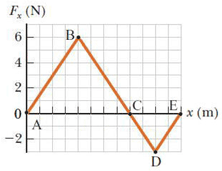# Problem: The force acting on a particle varies as in the figure below. (The x axis is marked in increments of 1.50 m.)    Find the work done by the force as the particle moves across the following distances.     from x = 0 m to x = 18.0 m

###### FREE Expert Solution

The work done is the sum of the two areas:###### Problem Details

The force acting on a particle varies as in the figure below. (The x axis is marked in increments of 1.50 m.)Find the work done by the force as the particle moves across the following distances.

from x = 0 m to x = 18.0 m